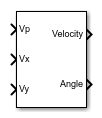# Resolver-to-Digital Converter

Resolver-to-digital converter

• Library:
• Simscape / Electrical / Control / Observers

•## Description

The Resolver-to-Digital Converter block models a transducer that converts the angular position or velocity of a rotating shaft to an electrical signal. Resolver-to-digital converters are commonly used in harsh, rugged environments, such as in fully electric vehicles.

The converted signal is proportional to the sine or cosine of the shaft angle.

A resolver sensor has one rotor winding with the exciter sine wave that is AC-coupled to two stator windings. The stator windings, a sine coil and a cosine coil, are mechanically positioned 90-degrees out-of-phase. As the rotor spins, the rotor position angle changes with respect to the stator windings. The resulting amplitude-modulated signals must then be gained, demodulated and post processed to extract angle and velocity information ( and ).

### Equations

The block uses a phase-locked loop (PLL) to extract the angle and the velocity of the rotating shaft. The error voltage used by the PI controller is obtained as:

`${V}_{e}={V}_{y}{V}_{p}\mathrm{cos}\left(N\theta \right)-{V}_{x}{V}_{p}\mathrm{sin}\left(N\theta \right),$`

where:

• `Vp` is the excitation voltage.

• `Vx` is the `x` voltage for the secondary winding of the resolver.

• `Vy` is the `y` voltage for the secondary winding of the resolver.

• `N` is the number of pole pairs for the resolver.

• `θ` is the angle.

Therefore, the velocity is obtained as:

`$\omega ={K}_{p}{V}_{e}+{K}_{i}\int {V}_{e},$`

and the angle is computed from the velocity using:

`$\frac{d\theta }{dt}=\omega .$`

## Ports

### Input

expand all

Simulink signal that corresponds to the voltage phase angle.

Data Types: `single` | `double`

Simulink signal that corresponds to the `x`-axis voltage.

Data Types: `single` | `double`

Simulink signal that corresponds to the `y`-axis voltage.

Data Types: `single` | `double`

### Output

expand all

Simulink signal that corresponds to the rotational velocity.

Data Types: `single` | `double`

Simulink signal that corresponds to the rotational angle.

Data Types: `single` | `double`

## Parameters

expand all

Number of pole pairs in the attached machine.

Proportional gain for the phase-locked loop filter. This value determines how aggressively the PLL tracks and locks to the phase angle. Increase this value to more closely track step changes in the phase angle.

Integral gain for the phase-locked loop filter. Increase this value to increase the rate at which steady-state error is eliminated in the phase angle. This value also determines how aggressively the PLL tracks and locks to the phase.

Initial estimate of the phase angle. If the input signal is a vector, use scalar parameters or use vector parameters that are the same size as the input signal.

Time between consecutive block executions. During execution, the block produces outputs and, if appropriate, updates its internal state. For more information, see What Is Sample Time? and Specify Sample Time.

For inherited discrete-time operation, specify `-1`. For discrete-time operation, specify a positive integer. For continuous-time operation, specify `0`.

Note

If this block is in a masked subsystem, or another variant subsystem that allows you to switch between continuous operation or discrete operation, promote the sample time parameter to ensure correct switching between the continuous and discrete implementations of the block. For more information, see Promote Block Parameters on a Mask.

 Santanu Sarma, V.K. Agrawal, Subramanya Udupa. Software-Based Resolver-to-Digital Conversion Using a DSP. IEEE Transactions on Industrial Electronics, 55, 371-379 . February 2008. (https://www.researchgate.net/publication/3219673_Software-Based_Resolver-to-Digital_Conversion_Using_a_DSP)

 Ankur Verma, Anand Chellamuthu. Design considerations for resolver-to-digital converters in electric vehicles. Texas Instruments, Analog Applications Journal. 2016.